How to build polyhedra with cardboard drawing polygons

This is a useful technique to build complex polyhedra. You do not need to draw the complete plane net. And our polyhedron can have different colors.You can download and print in different colors to build a lot of beautiful polyhedra:

Truncated TetrahedronThe truncated tetrahedron is an Archimedean solid made by 4 triangles and 4 hexagons.

Durer was the first to publish a plane net of a truncated tetrahedron: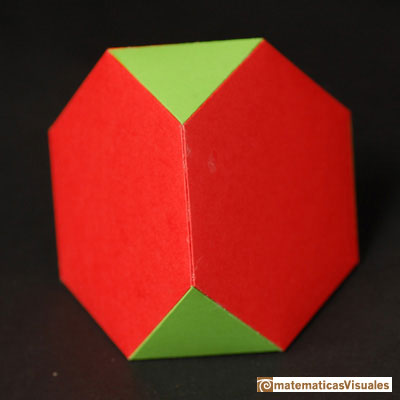CuboctahedronA cuboctahedron is an Archimedean solid. It can be seen as made by cutting off the corners of a cube.A cuboctahedron is an Archimedean solid. It can be seen as made by cutting off the corners of an octahedron.

It was Durer the first to publish plane nets of polyhedra. In his book 'Underweysung der Messung' ('Four Books of Measurement', published in 1525) the author draw plane developments of several Platonic and Archimedean solids, for example, this cuboctahedron: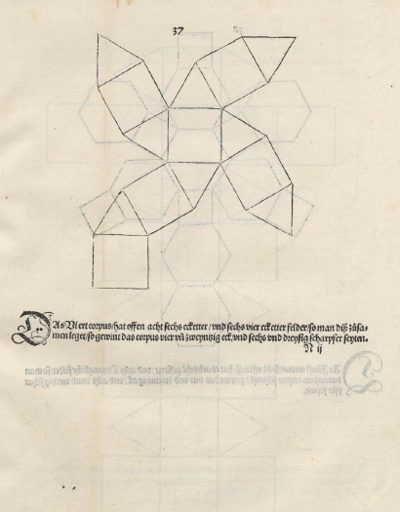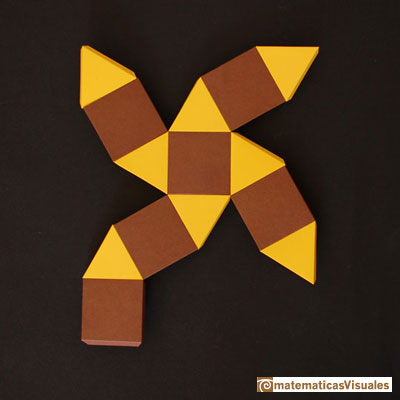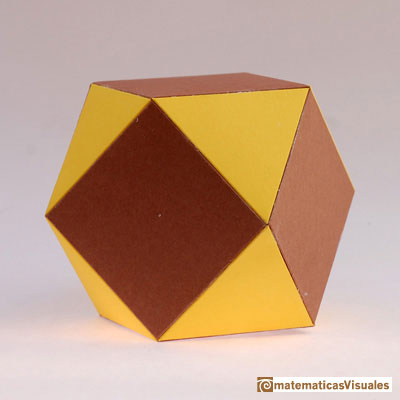Truncated OctahedronThe truncated octahedron is an Archimedean solid. It has 8 regular hexagonal faces and 6 square faces. Its volume can be calculated knowing the volume of an octahedron.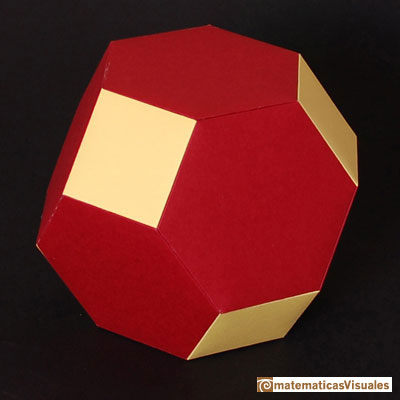This is the plane net of a truncated octahedron: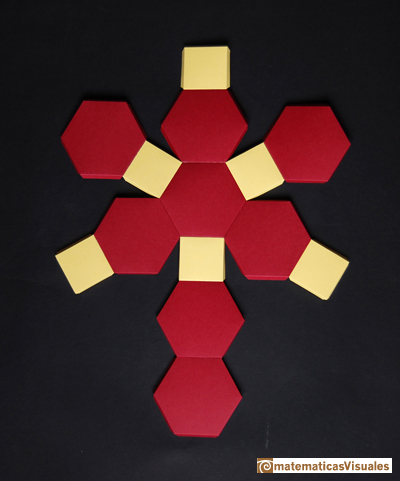More examplesI learned this technique in Wenninger's book. In those times I did not have easy access to a printer:REFERENCES

Magnus Wenninger - 'Polyhedron Models', Cambridge University Press.
Hugo Steinhaus - Mathematical Snapshots - Oxford University Press - Third Edition (p. 197)
Peter R. Cromwell - 'Polyhedra', Cambridge University Press, 1999.
H.Martin Cundy and A.P. Rollet, 'Mathematical Models', Oxford University Press, Second Edition, 1961 (p. 87).
W.W. Rouse Ball and H.S.M. Coxeter - 'Matematical Recreations & Essays', The MacMillan Company, 1947.Simple technique to build polyhedra gluing discs made of cardboard or paper.Italian designer Bruno Munari conceived 'Acona Biconbi' as a work of sculpture. It is also a beautiful game to play with colors and shapes.Material for a session about polyhedra (Zaragoza, 9th May 2014). Simple techniques to build polyhedra like the tetrahedron, octahedron, the cuboctahedron and the rhombic dodecahedron. We can build a box that is a rhombic dodecahedron.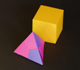Material for a session about polyhedra (Zaragoza, 7th November 2014). We study the octahedron and the tetrahedron and their volumes. The truncated octahedron helps us to this task. We build a cubic box with cardboard and an origami tetrahedron.Material for a session about polyhedra (Zaragoza, 23rd Octuber 2015) . Building a cube with cardboard and an origami octahedron.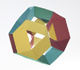Material for a session about polyhedra (Zaragoza, 21st October 2016). Instructions to build several geometric bodies.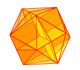The twelve vertices of an icosahedron lie in three golden rectangles. Then we can calculate the volume of an icosahedronThe volume of a tetrahedron is one third of the prism that contains it.The first drawing of a plane net of a regular tetrahedron was published by Dürer in his book 'Underweysung der Messung' ('Four Books of Measurement'), published in 1525 .Some properties of this platonic solid and how it is related to the golden ratio. Constructing dodecahedra using different techniques.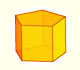We study different prisms and we can see how they develop into a plane net. Then we explain how to calculate the lateral surface area.We study different cylinders and we can see how they develop into a plane. Then we explain how to calculate the lateral surface area.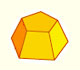Plane net of pyramids and pyramidal frustrum. How to calculate the lateral surface area.Plane developments of cones and conical frustum. How to calculate the lateral surface area.The first drawing of a plane net of a regular dodecahedron was published by Dürer in his book 'Underweysung der Messung' ('Four Books of Measurement'), published in 1525 .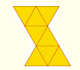The first drawing of a plane net of a regular octahedron was published by Dürer in his book 'Underweysung der Messung' ('Four Books of Measurement'), published in 1525 .The first drawing of a plane net of a regular tetrahedron was published by Dürer in his book 'Underweysung der Messung' ('Four Books of Measurement'), published in 1525 .